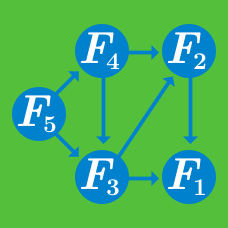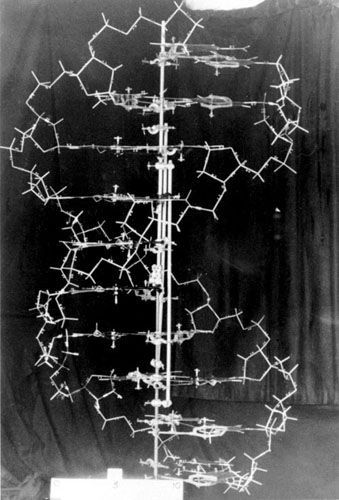Computer Science

# Dynamic Programming - Problem SolvingSuppose we are given two short DNA sequences seq1 and seq2.Suppose we are also given a set of operations:

• Replace : Replace one character of a string with any other character.
• Insert : Insert one character into a string.
• Delete: Remove one character into another string.

Consider the two sequences below

 1 2 seq1 = 'CTCCCAGGGTG' seq2 = 'ACGTATAGCTTG' 

What is the minimum number of operations required to convert one sequence into the other?

Brilli the ant sees a square $n \times n$ matrix consisting of only $0$'s and $1$s. He is given the task of finding the largest $1$ square space( the largest square submatrix consisting of only $1$'s) . For example if he was given the below table, the shaded area would be the largest square area:Unfortunately Brilli is given a matrix of much larger size. Being the competent programmer that he is, he divides the problem into subproblems and comes up with a brilliant dynamic programming solution.

His dynamic programming solution considers the subproblem $S[i,j]$. $S[i,j]$ being the side length of the largest $1$ square space whose bottom-right corner is at $i,j$.

Once he solves the problem, his table has the following values:

$S = 4$ $S = 10$ $S = 12$

Given that there is a 1 at , what is the value of $S$?

The longest common subsequence (LCS) problem is defined as follows:

Given two strings: string $S$ of length $n$ and string $T$ of length $m$, the goal is to produce their longest common subsequence: the longest sequence of characters that appear left-to-right (but not necessarily in a contiguous block) in both strings.

For example:

$S$= BZHAC $T$= HKABFT

Then the longest common subsequence has a length of $3$ and is HAB.

Suppose you have the following snippets of two genes which you suspect to code for homologous proteins, but to have diverged long ago on the evolutionary scale. Find their LCS, what is its length?

  1 2 3 4 5 6 7 8 9 10 11 12 sequence_1 = GGCAAGGTACTTCCGGTCTTAATGAATGGCCGGG AAAGGTACGCACGCGGTATGGGGGGGTGAAGGGGCGAATAGACAGGC TCCCCTCTCACTCGCTAGGAGGCAATTGTATAAGAATGCATACTGCA TCGATACATAAAACGTCTCCATCGCTTGCCCAAGTTGTGAAGTGTCT ATCACCCCTAGGCCCGTTTCCCGCA sequence_2 = GGCTGGCGTTTTGAATCCTCGGTCCCCCTTGTCT ATCCAGATTAATCCAATTCCCTCATTTAGGACCCTACCAAGTCAACA TTGGTATATGAATGCGACCTCGAAGAGGCCGCCTAAAAATGACAGTG GTTGGTGCTCTAAACTTCATTTGGTTAACTCGTGTATCAGCGCGATA GGCTGTTAGAGGTTTAATATTGTAT 

×